Скачать презентацию Risk and return 1 Class 9 Financial Management

e4e3cc024b1244a914c2cb1a1c860840.ppt

• Количество слайдов: 39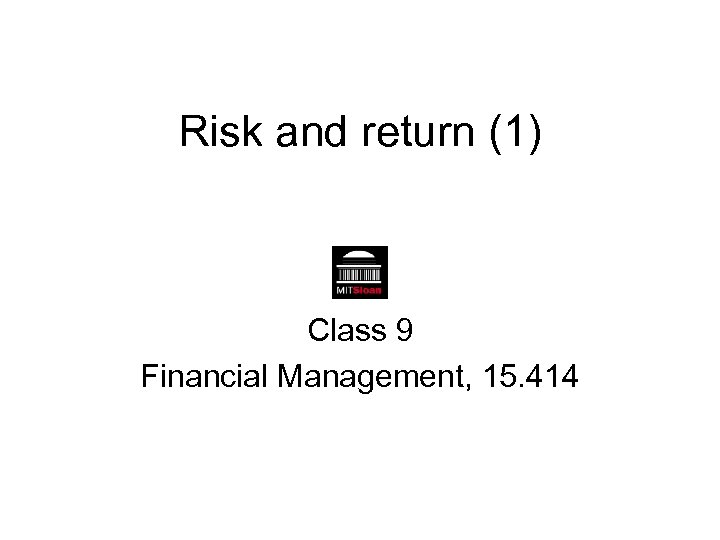Risk and return (1) Class 9 Financial Management, 15. 414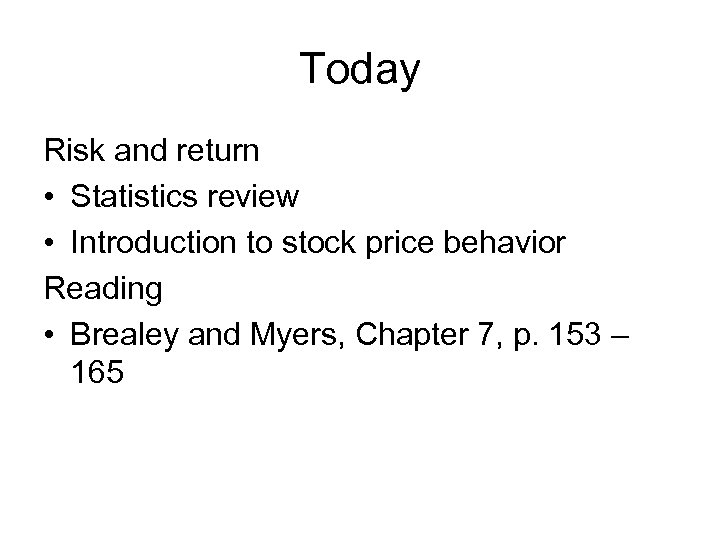Today Risk and return • Statistics review • Introduction to stock price behavior Reading • Brealey and Myers, Chapter 7, p. 153 – 165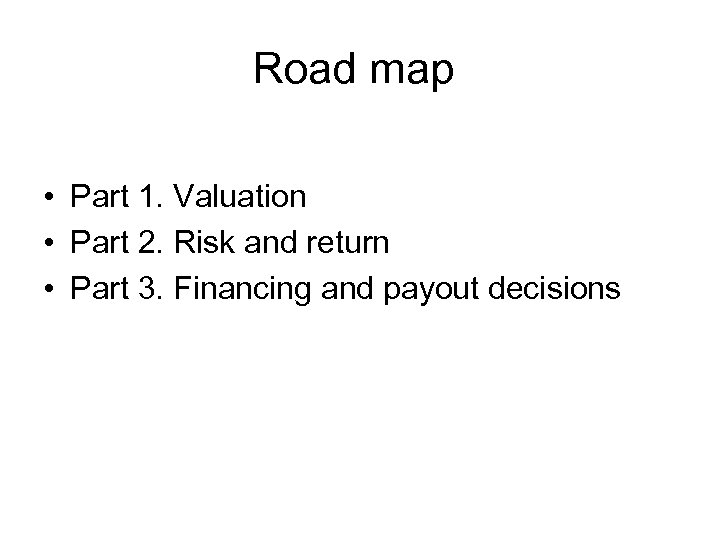Road map • Part 1. Valuation • Part 2. Risk and return • Part 3. Financing and payout decisions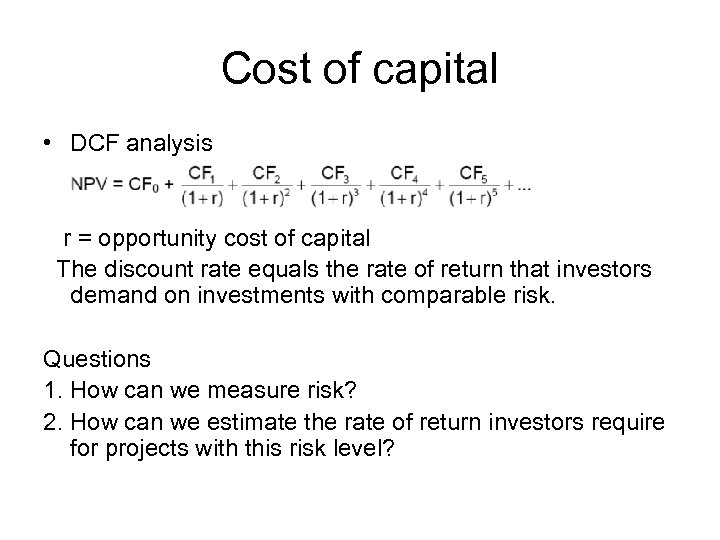Cost of capital • DCF analysis r = opportunity cost of capital The discount rate equals the rate of return that investors demand on investments with comparable risk. Questions 1. How can we measure risk? 2. How can we estimate the rate of return investors require for projects with this risk level?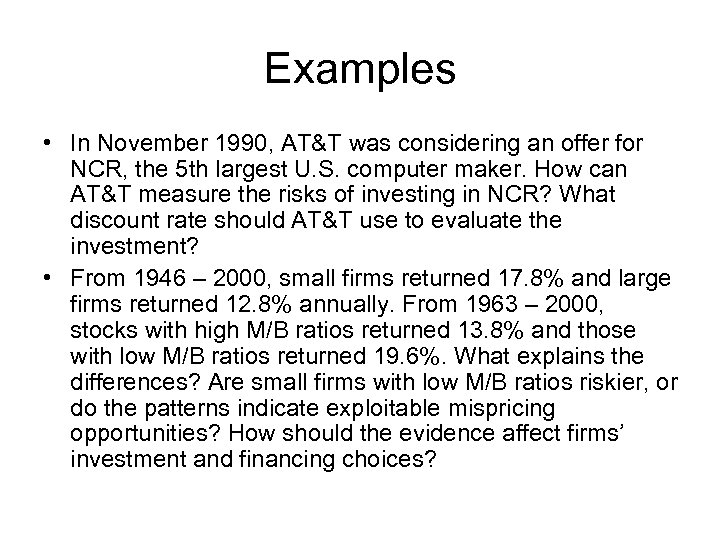Examples • In November 1990, AT&T was considering an offer for NCR, the 5 th largest U. S. computer maker. How can AT&T measure the risks of investing in NCR? What discount rate should AT&T use to evaluate the investment? • From 1946 – 2000, small firms returned 17. 8% and large firms returned 12. 8% annually. From 1963 – 2000, stocks with high M/B ratios returned 13. 8% and those with low M/B ratios returned 19. 6%. What explains the differences? Are small firms with low M/B ratios riskier, or do the patterns indicate exploitable mispricing opportunities? How should the evidence affect firms’ investment and financing choices?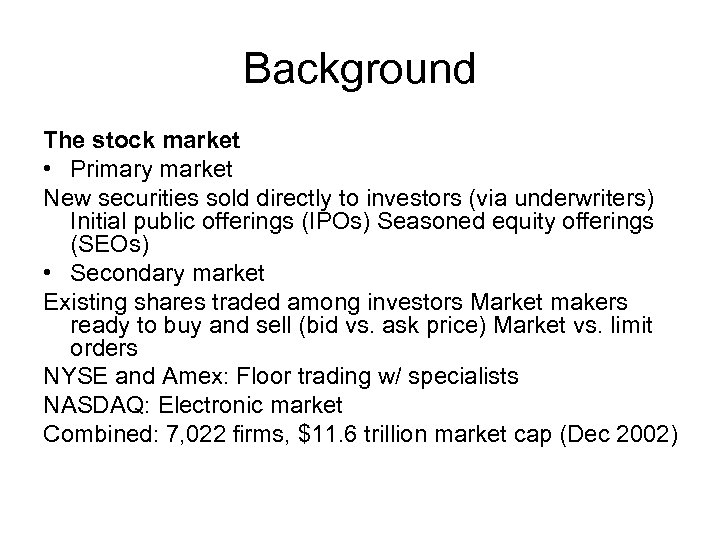Background The stock market • Primary market New securities sold directly to investors (via underwriters) Initial public offerings (IPOs) Seasoned equity offerings (SEOs) • Secondary market Existing shares traded among investors Market makers ready to buy and sell (bid vs. ask price) Market vs. limit orders NYSE and Amex: Floor trading w/ specialists NASDAQ: Electronic market Combined: 7, 022 firms, \$11. 6 trillion market cap (Dec 2002)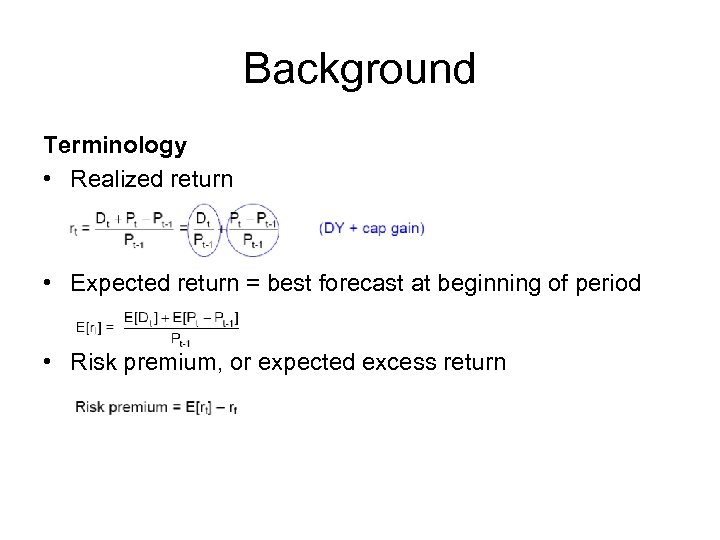Background Terminology • Realized return • Expected return = best forecast at beginning of period • Risk premium, or expected excess return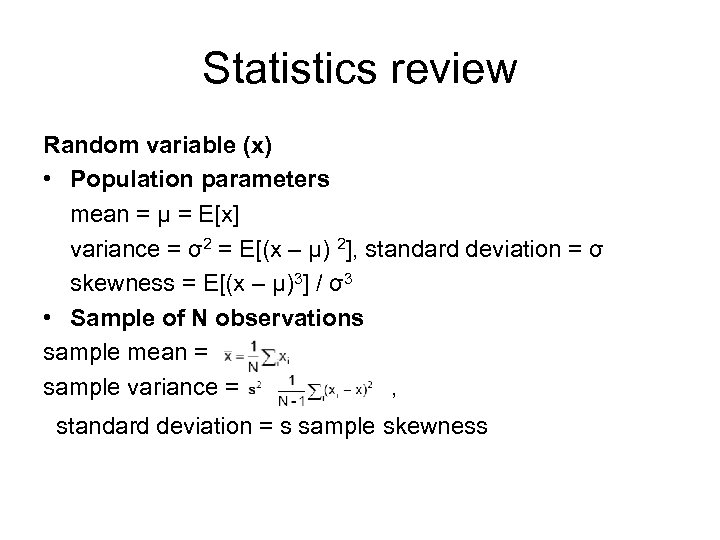Statistics review Random variable (x) • Population parameters mean = μ = E[x] variance = σ2 = E[(x – μ) 2], standard deviation = σ skewness = E[(x – μ)3] / σ3 • Sample of N observations sample mean = sample variance = , standard deviation = s sample skewness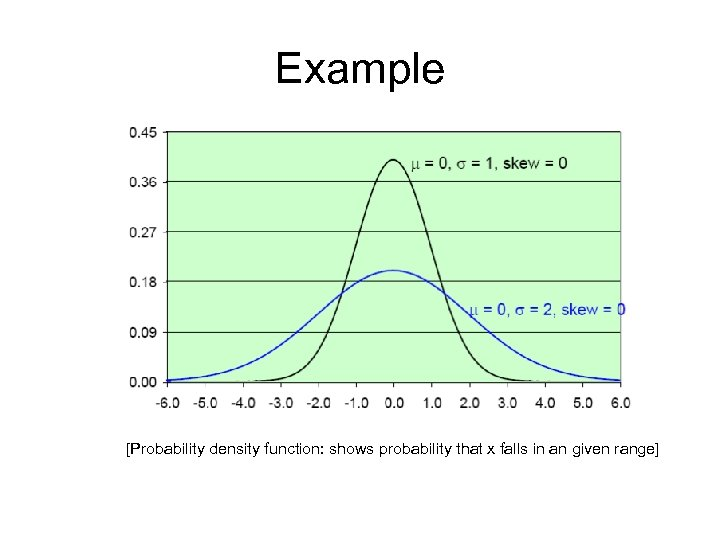Example [Probability density function: shows probability that x falls in an given range]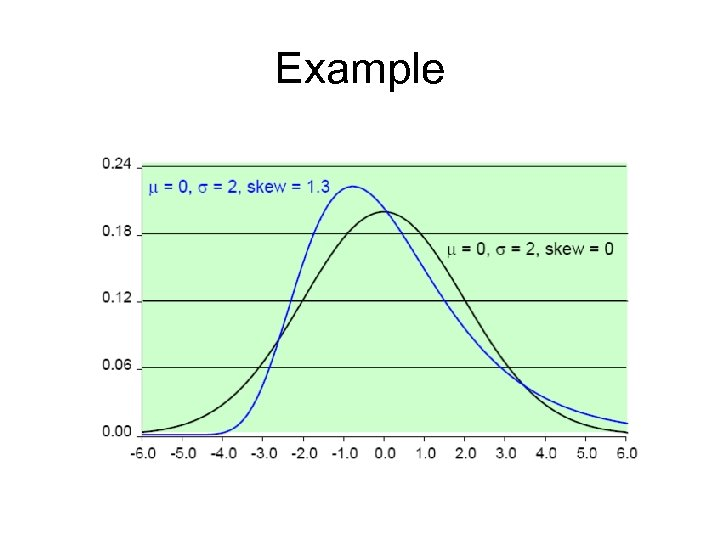Example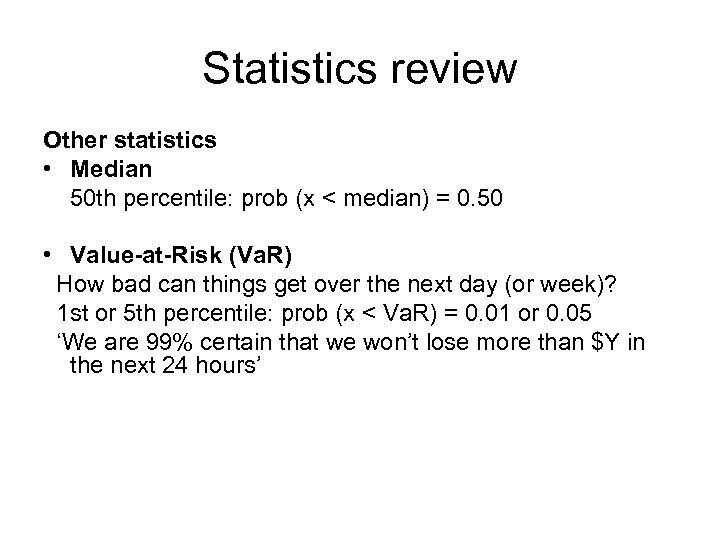Statistics review Other statistics • Median 50 th percentile: prob (x < median) = 0. 50 • Value-at-Risk (Va. R) How bad can things get over the next day (or week)? 1 st or 5 th percentile: prob (x < Va. R) = 0. 01 or 0. 05 ‘We are 99% certain that we won’t lose more than \$Y in the next 24 hours’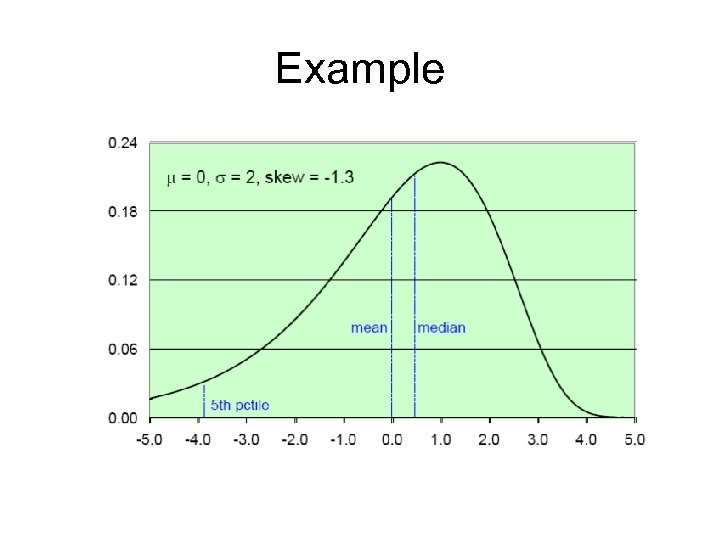Example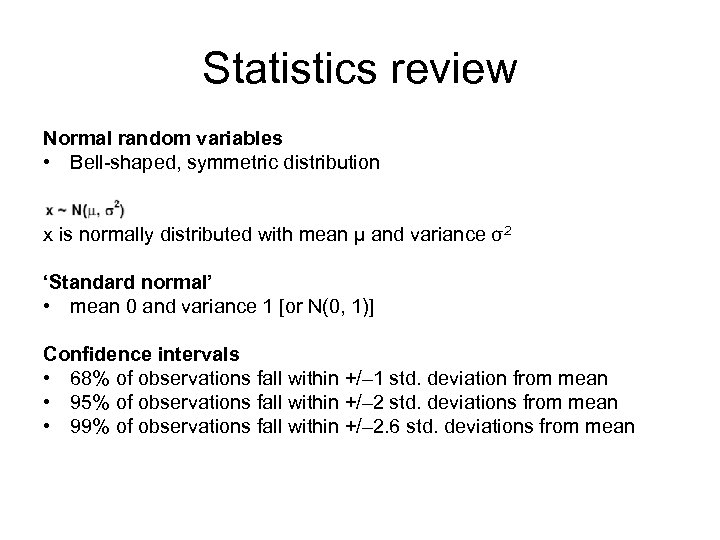Statistics review Normal random variables • Bell-shaped, symmetric distribution x is normally distributed with mean μ and variance σ2 ‘Standard normal’ • mean 0 and variance 1 [or N(0, 1)] Confidence intervals • 68% of observations fall within +/– 1 std. deviation from mean • 95% of observations fall within +/– 2 std. deviations from mean • 99% of observations fall within +/– 2. 6 std. deviations from mean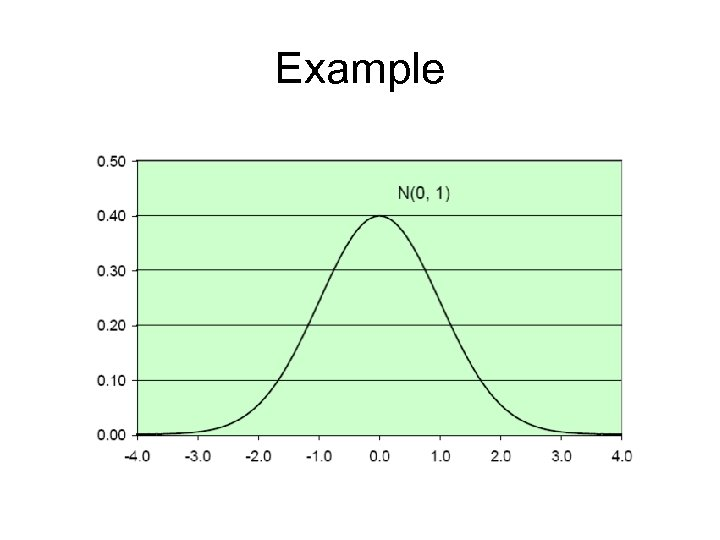Example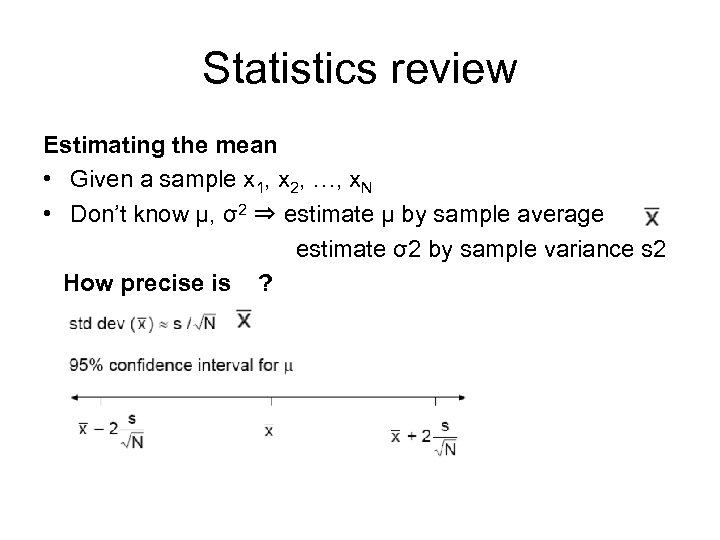Statistics review Estimating the mean • Given a sample x 1, x 2, …, x. N • Don’t know μ, σ2 ⇒ estimate μ by sample average estimate σ2 by sample variance s 2 How precise is ?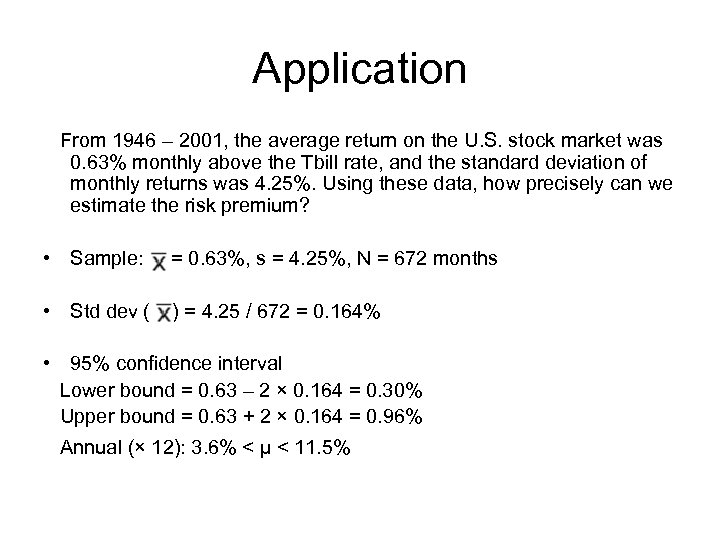Application From 1946 – 2001, the average return on the U. S. stock market was 0. 63% monthly above the Tbill rate, and the standard deviation of monthly returns was 4. 25%. Using these data, how precisely can we estimate the risk premium? • Sample: = 0. 63%, s = 4. 25%, N = 672 months • Std dev ( ) = 4. 25 / 672 = 0. 164% • 95% confidence interval Lower bound = 0. 63 – 2 × 0. 164 = 0. 30% Upper bound = 0. 63 + 2 × 0. 164 = 0. 96% Annual (× 12): 3. 6% < μ < 11. 5%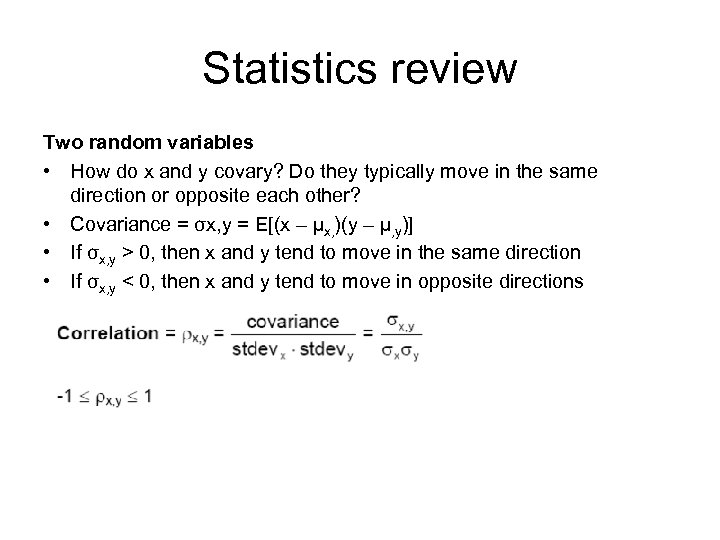Statistics review Two random variables • How do x and y covary? Do they typically move in the same direction or opposite each other? • Covariance = σx, y = E[(x – μx, )(y – μ, y)] • If σx, y > 0, then x and y tend to move in the same direction • If σx, y < 0, then x and y tend to move in opposite directions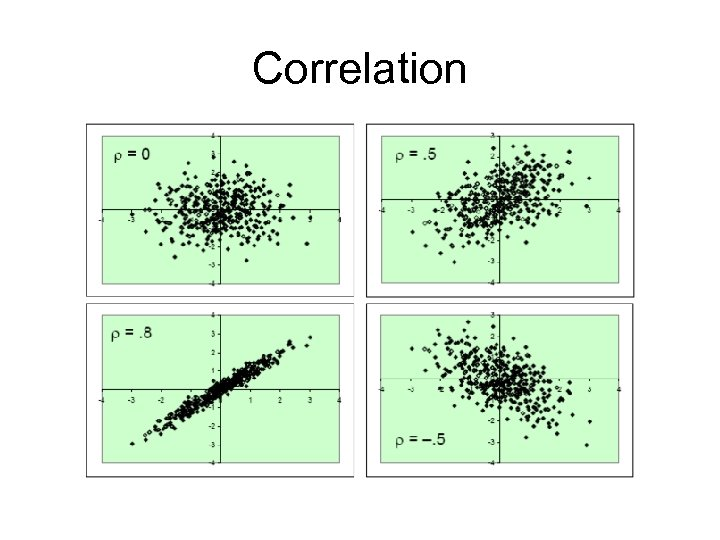Correlation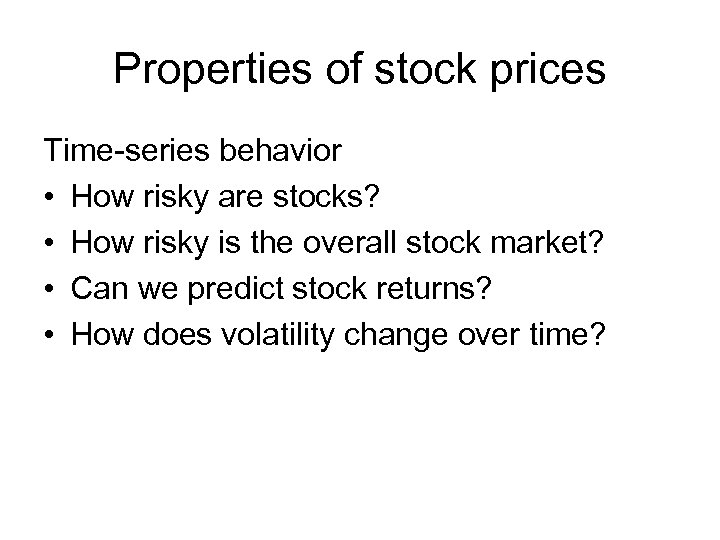Properties of stock prices Time-series behavior • How risky are stocks? • How risky is the overall stock market? • Can we predict stock returns? • How does volatility change over time?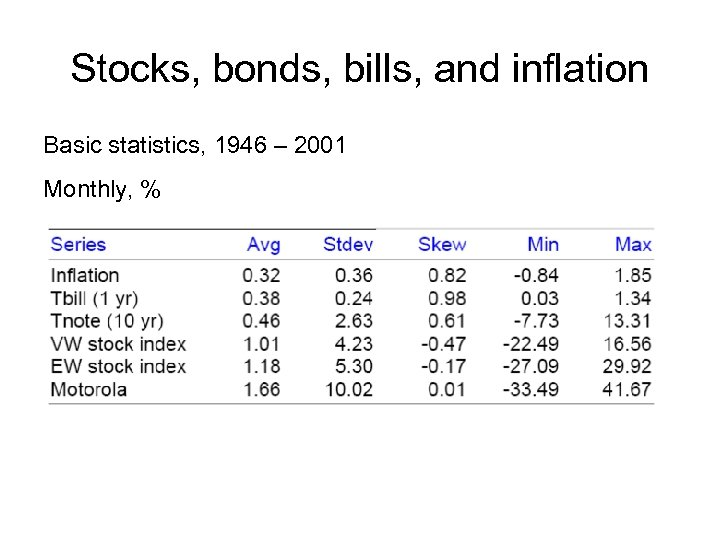Stocks, bonds, bills, and inflation Basic statistics, 1946 – 2001 Monthly, %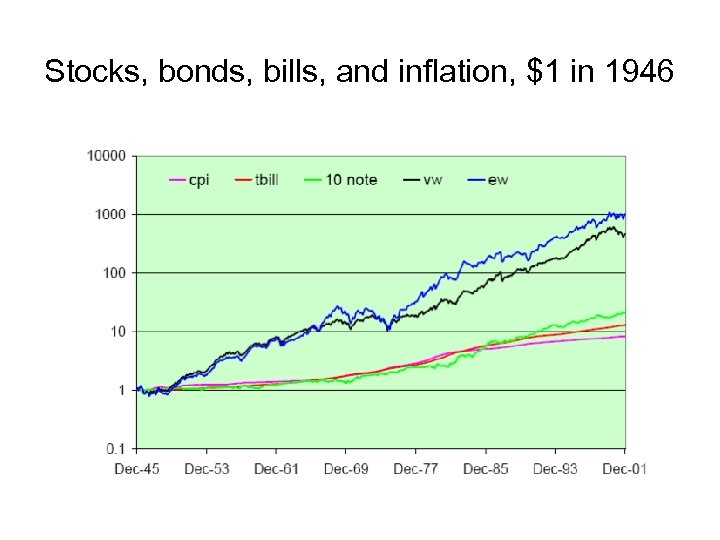Stocks, bonds, bills, and inflation, \$1 in 1946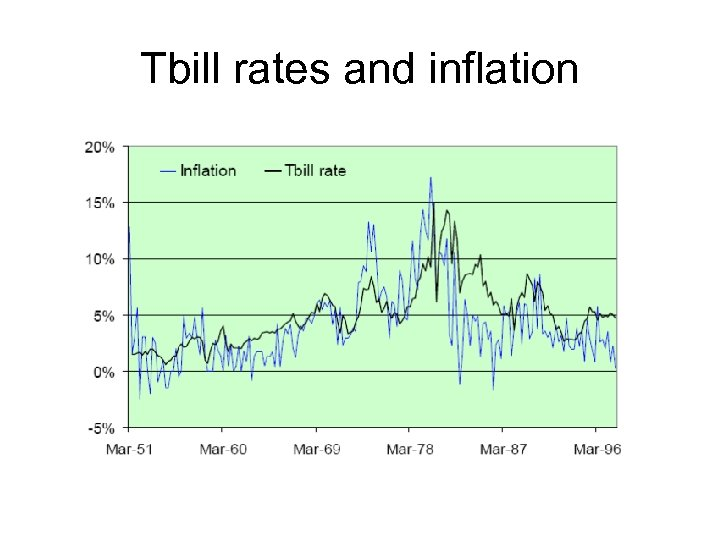Tbill rates and inflation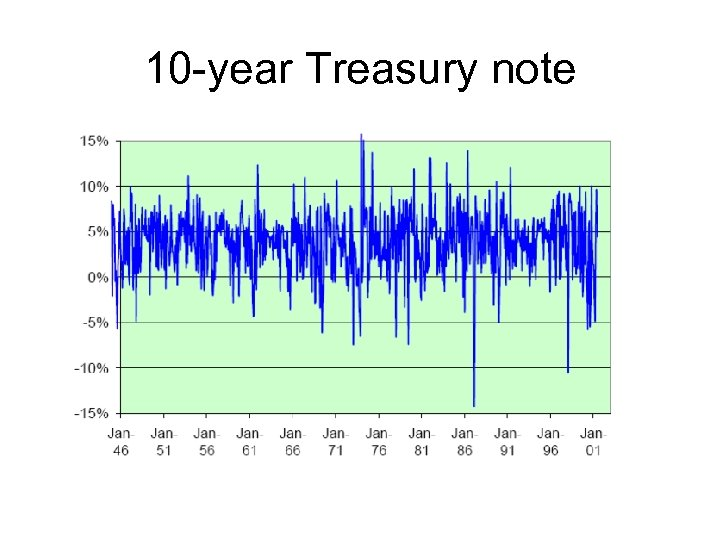10 -year Treasury note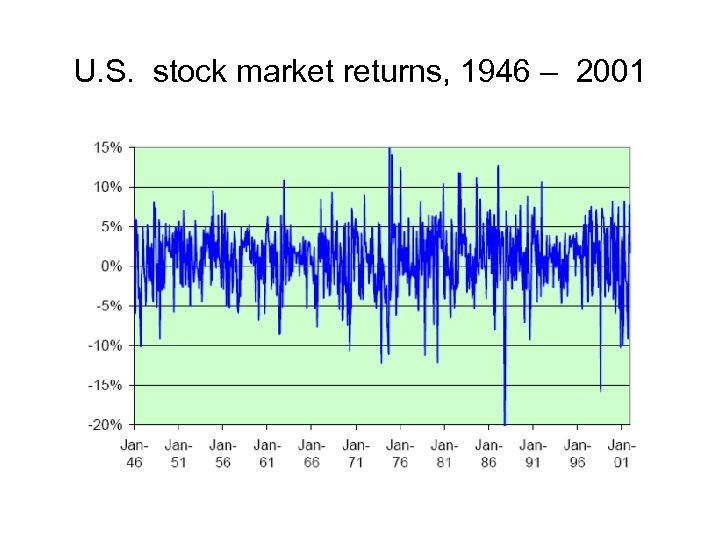U. S. stock market returns, 1946 – 2001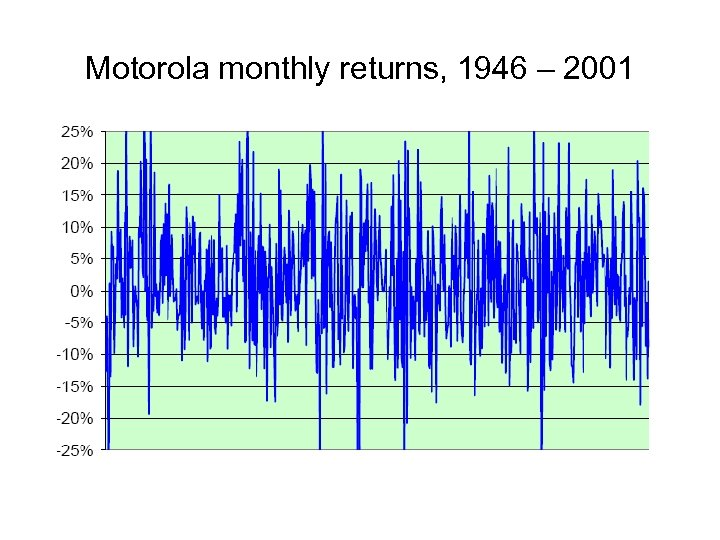Motorola monthly returns, 1946 – 2001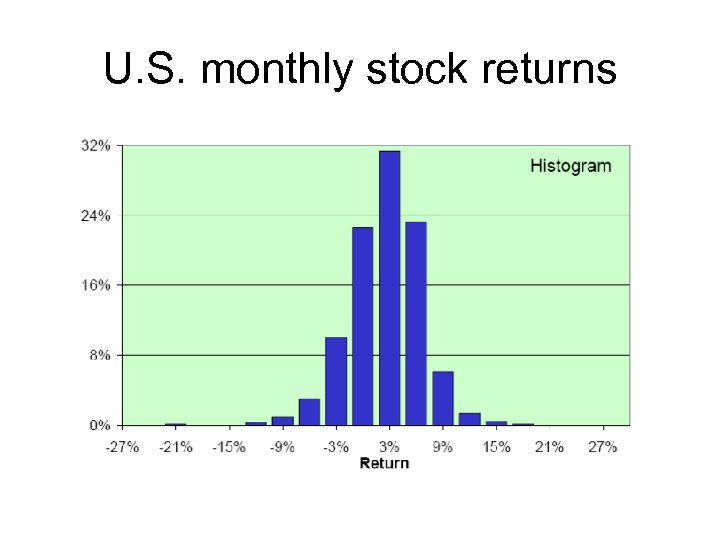U. S. monthly stock returns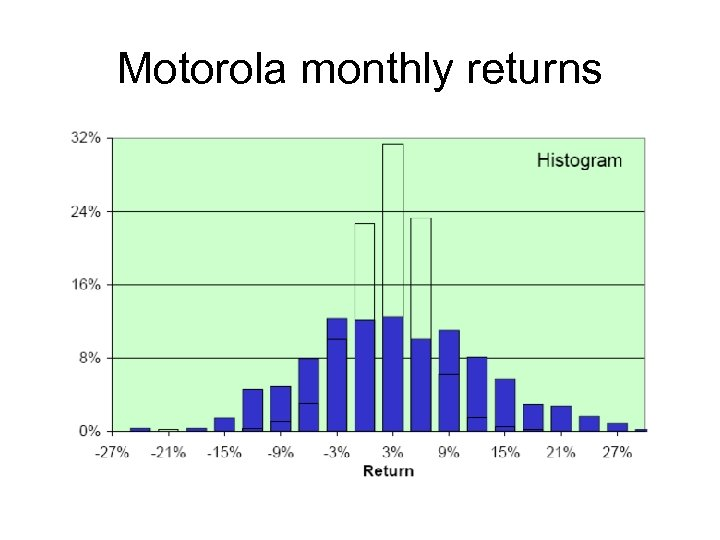Motorola monthly returns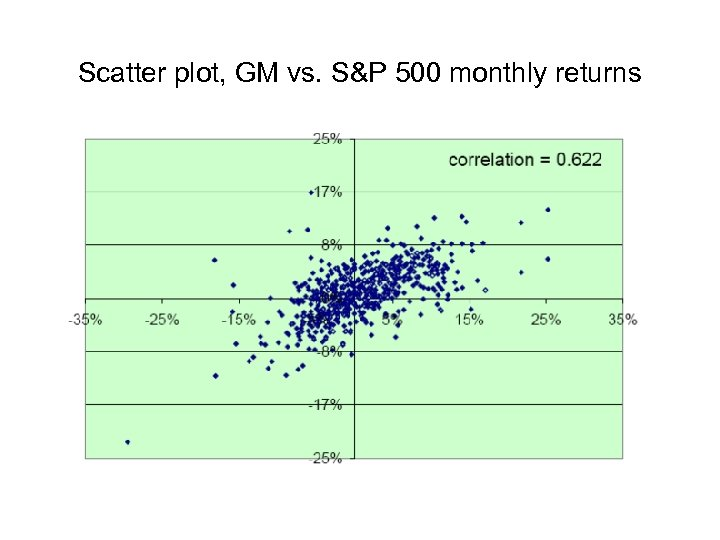Scatter plot, GM vs. S&P 500 monthly returns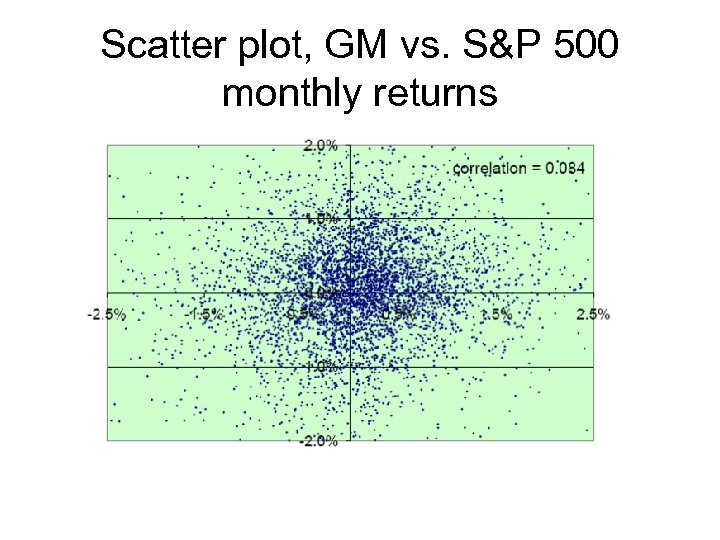Scatter plot, GM vs. S&P 500 monthly returns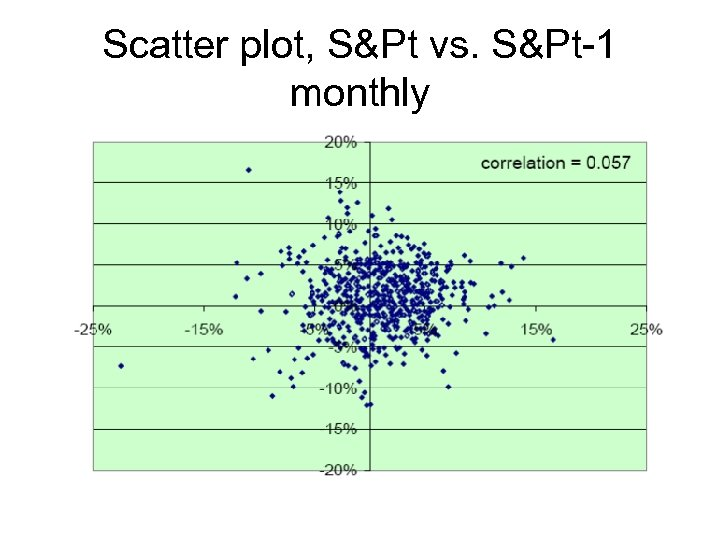Scatter plot, S&Pt vs. S&Pt-1 monthly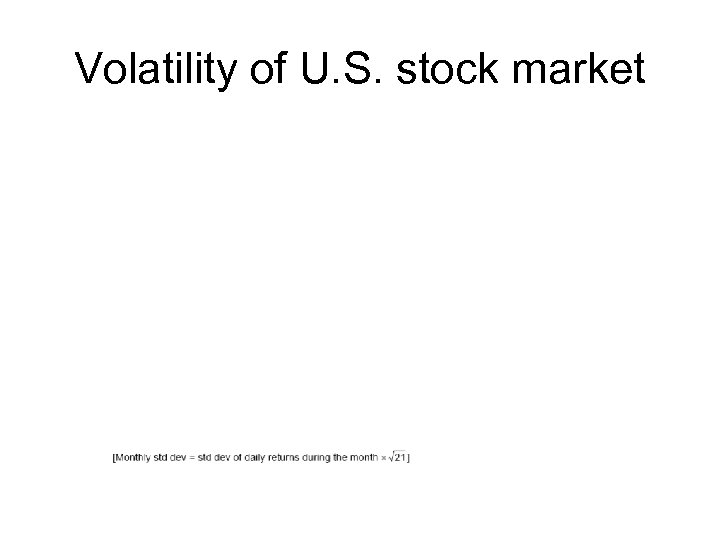Volatility of U. S. stock market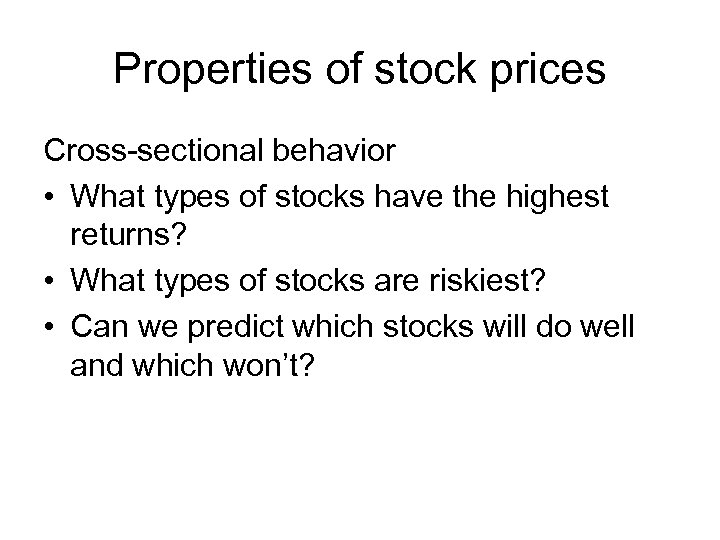Properties of stock prices Cross-sectional behavior • What types of stocks have the highest returns? • What types of stocks are riskiest? • Can we predict which stocks will do well and which won’t?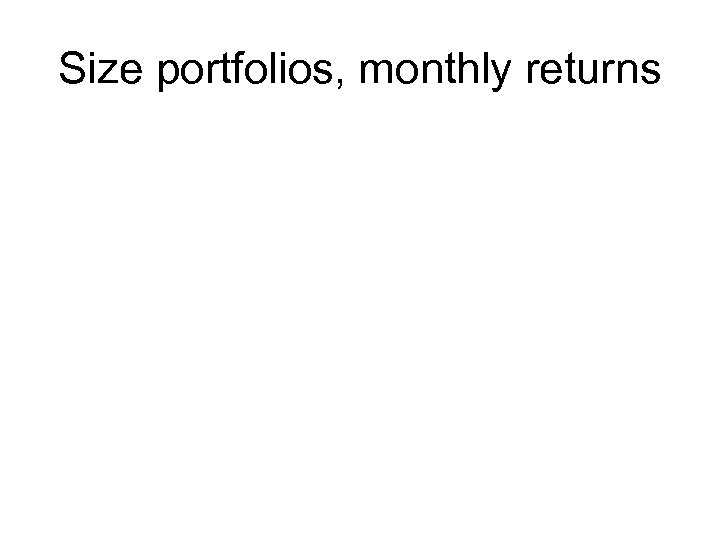Size portfolios, monthly returns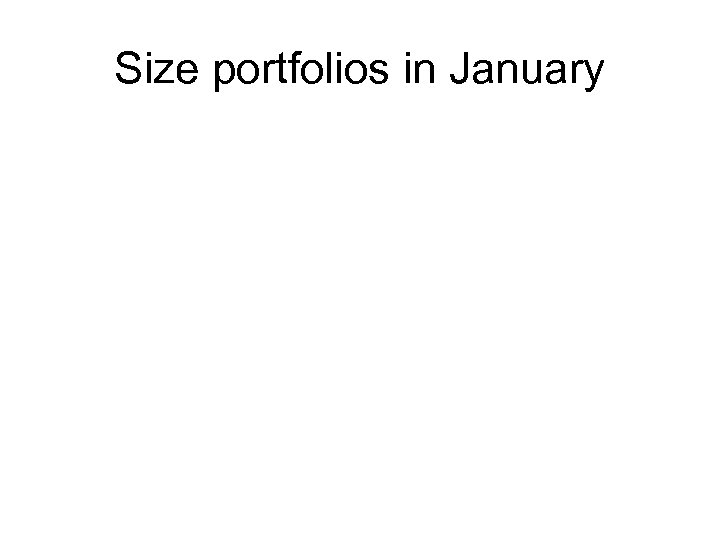Size portfolios in January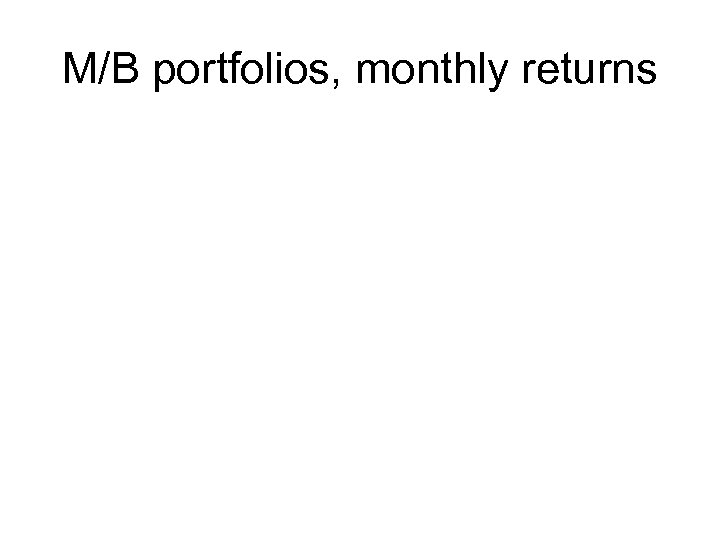M/B portfolios, monthly returns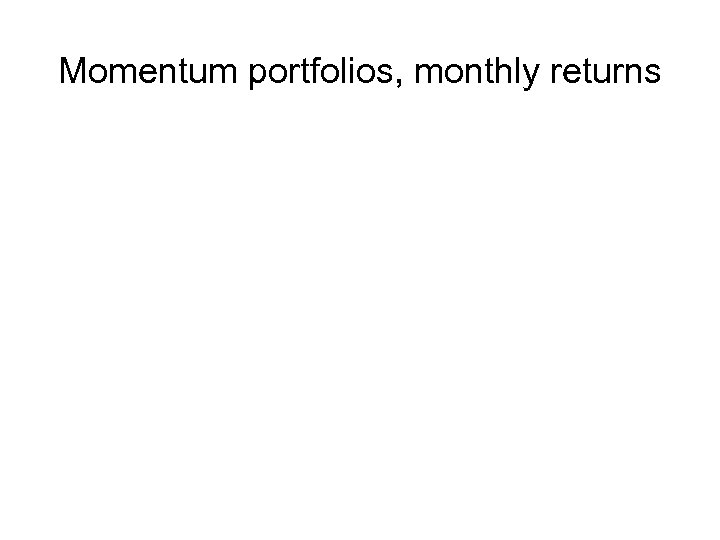Momentum portfolios, monthly returns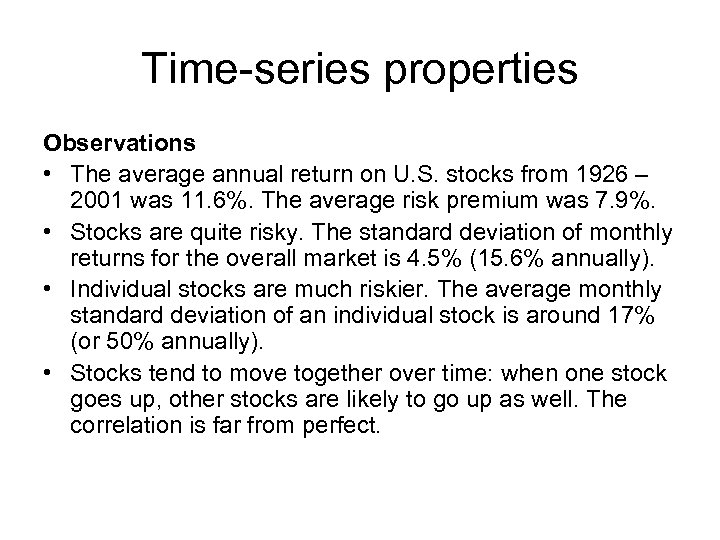Time-series properties Observations • The average annual return on U. S. stocks from 1926 – 2001 was 11. 6%. The average risk premium was 7. 9%. • Stocks are quite risky. The standard deviation of monthly returns for the overall market is 4. 5% (15. 6% annually). • Individual stocks are much riskier. The average monthly standard deviation of an individual stock is around 17% (or 50% annually). • Stocks tend to move together over time: when one stock goes up, other stocks are likely to go up as well. The correlation is far from perfect.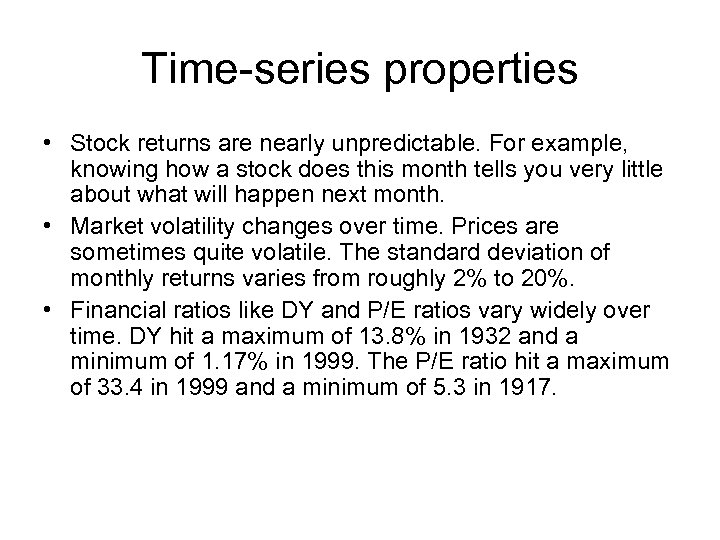Time-series properties • Stock returns are nearly unpredictable. For example, knowing how a stock does this month tells you very little about what will happen next month. • Market volatility changes over time. Prices are sometimes quite volatile. The standard deviation of monthly returns varies from roughly 2% to 20%. • Financial ratios like DY and P/E ratios vary widely over time. DY hit a maximum of 13. 8% in 1932 and a minimum of 1. 17% in 1999. The P/E ratio hit a maximum of 33. 4 in 1999 and a minimum of 5. 3 in 1917.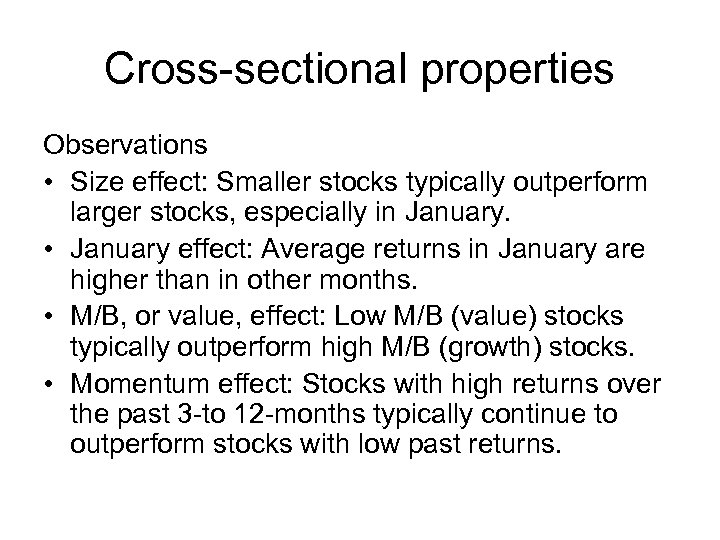Cross-sectional properties Observations • Size effect: Smaller stocks typically outperform larger stocks, especially in January. • January effect: Average returns in January are higher than in other months. • M/B, or value, effect: Low M/B (value) stocks typically outperform high M/B (growth) stocks. • Momentum effect: Stocks with high returns over the past 3 -to 12 -months typically continue to outperform stocks with low past returns.